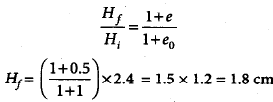Courses

# Test: Geotechnical Engineering- 1

## 15 Questions MCQ Test GATE Civil Engineering (CE) 2022 Mock Test Series | Test: Geotechnical Engineering- 1

Description
This mock test of Test: Geotechnical Engineering- 1 for GATE helps you for every GATE entrance exam. This contains 15 Multiple Choice Questions for GATE Test: Geotechnical Engineering- 1 (mcq) to study with solutions a complete question bank. The solved questions answers in this Test: Geotechnical Engineering- 1 quiz give you a good mix of easy questions and tough questions. GATE students definitely take this Test: Geotechnical Engineering- 1 exercise for a better result in the exam. You can find other Test: Geotechnical Engineering- 1 extra questions, long questions & short questions for GATE on EduRev as well by searching above.
QUESTION: 1

### Which one of the following relations is not correct?

Solution:

The correct relations are as follows:
e=n/(1-n)
γs=((G+e)/(1+e)) γw
n=e/(1+e)
e=wG/S

QUESTION: 2

### The void ratio-pressure diagram is shown in figure given below. The coefficient of compressibility is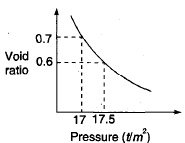Solution: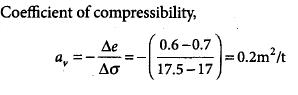QUESTION: 3

### Standard Newmark’s influence chart is shown in the given figure. if loaded equally, the areas marked 1 and 2 will yield pressures at the center such that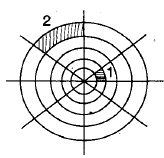Solution:

Any subarea of Newmark’s chart has same influence value. Therefore, 1 and 2 will yield same pressure at the center.

QUESTION: 4

A saturates soil mass has a total density 22 kN/m3 and a water content of 10%. The bulk density and dry density of this soil are

Solution:

For saturated soil,

γbulk = γsat = 22 kN/m3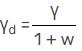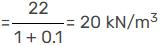QUESTION: 5

The factor of safety of a slope of infinite extent having a slope angle = 25°, and the slope is made of cohensionless soil with φ = 30° is.

Solution: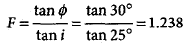QUESTION: 6

In a three-layered soil, water flows parallel to stratification. The thickness of the middle layer is twice that of the top and bottom layer. The coefficient of permeability of middle layer (2K) is twice that of top and bottom layer (K). What is the average coefficient of permeability for this flow?

Solution: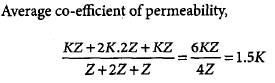QUESTION: 7

The dry density of a soil is 1.5 g/cc. if the saturation water content is 50%, then its saturated density and submerged density would, respectively, be

Solution: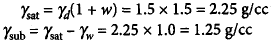QUESTION: 8

A soil has a bulk density of 1.80 g/cm3 at water content of 5%. If the void ratio remains constant, then its bulk density for the water content of 10% will be

Solution: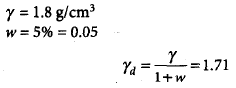Voids ratio does not change after drying. Therefore, γd remains same.
γ = γd(1 + w) = 1.71(1 + 0.1) = 1.88 g/cm2

QUESTION: 9

____________ is the measure of loss of strength with remoulding, with water content unchanged.

Solution:

Sensitivity values ranges from 1-16. For an insensitive soil, it is 1. A soil with sensitivity above 16, is termed as quick.

QUESTION: 10

In Geotechnical Engineering, soil is considered as a _________ phase material.

Solution:

Soil is a 3 phase material. It consists of solids, water and air voids. It is represented in a 3 phase diagram with volume on left side and mass on the right side.

*Answer can only contain numeric values
QUESTION: 11

In a plate load test on sandy soil, the test plate of 60 cm × 60 cm undergoes a settlement of 5 mm at a pressure of 12 × 104 N/m2. The expected settlement of 3 m × 3 m footing under same pressure will be _________ mm.

Solution:

Sf = Sp* { Bf (Bp + .3)/Bp (Bf+ .3) }2
= 5 * { 3 ( .6+ .3)/ .6 ( 3+ .3)}2
= 9.24 mm.

QUESTION: 12

A normally consolidated clay layer settles by 25 mm when the effective stress is increased from 15 to 30 kPa. If the effective stress is later increased further from 30 kPa to 60 kPa, then the additional settlement would be

Solution: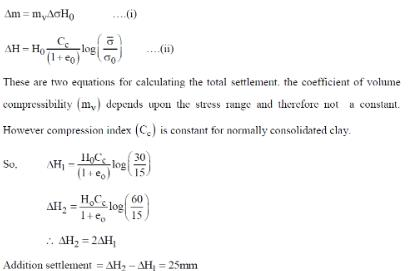QUESTION: 13

A flownet constructed to determine the seepage through an earth dam which is homogenous but anisotropic, gave four flow channels and sixteen equipotential drops. The coefficients of permeability in the horizontal and vertical directions are 4 × 10–7 m/s and 1.0 × 10–7 m/s, respectively. If the storage head was 20 m, then the seepage per unit length of the dam (in m3/s) would be

Solution: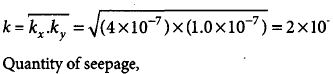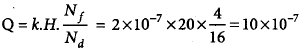*Answer can only contain numeric values
QUESTION: 14

A clay soil specimen tested in unconfined condition gave an unconfined compressive strength of 100 kN/m2. A specimen of the same clay with the same initial condition is subjected to a UU triaxial test under a cell pressure of 100 kN/m2. The axial stress (in kN/m2) at failure would be ____________.

Solution: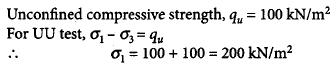QUESTION: 15

The initial and final void ratios of a clay sample in a consolidation  test are 1 and 0.5, respectively. If the initial thickness of the sample is 2.4 cm, then its final thickness will be

Solution: